# Surface area of a sphere problems

#### Number of problems found: 41

• Sphere in coneA sphere is inscribed in the cone (the intersection of their boundaries consists of a circle and one point). The ratio of the surface of the ball and the contents of the base is 4: 3. A plane passing through the axis of a cone cuts the cone in an isosceleHow many times does the surface of a sphere decrease if we reduce its radius twice?
• EightEight small Christmas balls with a radius of 1 cm have the same volume as one large Christmas ball. What has a bigger surface: eight small balls, or one big ball?
• Hemisphere - roof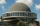The shape of the observatory dome is close to the hemisphere. Its outer diameter is 11 m. How many kilograms of paint and how many liters of thinner is used for its double coat if you know that 1 kg of paint diluted with 1 deciliter of thinner will paint
• The ballThe ball has a radius of 2m. What percentage of the surface and volume is another sphere whose radius is 20% larger?
• Sphere VSFind the surface and volume of a sphere that has a radius of 2 dm.The radius of the sphere we reduce by 1/3 of the original radius. How much percent does the volume and surface of the sphere change?
• Spherical capPlace a part of the sphere on a 4.6 cm cylinder so that the surface of this section is 20 cm2. Determine the radius r of the sphere from which the spherical cap was cut.
• Half-sphere roofThe roof above the castle tower has the shape of a 12.8 m diameter half-sphere. What is the cost of this roof, if the cost of 1 square meter is 12 euros and 40 cents?
• Iron ball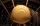The iron ball has a weight of 100 kilograms. Calculate the volume, radius, and surface if the iron's density is h = 7.6g/cm3.
• The observatoryThe dome of the hemisphere-shaped observatory is 5.4 meters high. How many square meters of sheet metal needs to be covered to cover it, and 15 percent must be added to the minimum amount due to joints and waste?
• PilotHow high is the airplane's pilot to see 0.001 of Earth's surface?
• Tropical, mild and arctic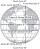How many percents of the Earth's surface lies in the tropical, mild and arctic range? The border between the ranges is the parallel 23°27 'and 66°33'.The surface of the sphere is 60 cm square. Calculate its radius; result round to tenth of cm.
• Spheres in sphere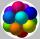How many spheres with a radius of 15 cm can fits into the larger sphere with a radius of 150 cm?
• Sphere vs cubeHow many % of the surface of a sphere of radius 12 cm is the surface of a cube inscribed in this sphere?
• Above EarthTo what height must a boy be raised above the earth in order to see one-fifth of its surface.
• AirplaneAviator sees part of the earth's surface with an area of 200,000 square kilometers. How high he flies?
• Fit ballWhat is the size of the surface of Gymball (FIT - ball) with a diameter of 65 cm?
• Cube from sphere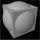What largest surface area (in cm2) can have a cube that was cut out of a sphere with radius 43 cm?

Do you have an interesting mathematical word problem that you can't solve it? Submit a math problem, and we can try to solve it.

We will send a solution to your e-mail address. Solved examples are also published here. Please enter the e-mail correctly and check whether you don't have a full mailbox.

Please do not submit problems from current active competitions such as Mathematical Olympiad, correspondence seminars etc...